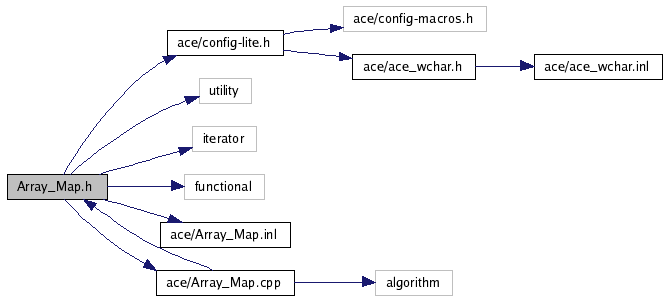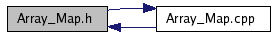# Array_Map.h File Reference

`#include "ace/config-lite.h"`
`#include <utility>`
`#include <iterator>`
`#include <functional>`
`#include "ace/Array_Map.inl"`
`#include "ace/Array_Map.cpp"`

Include dependency graph for Array_Map.h:This graph shows which files directly or indirectly include this file:## Classes

class  ACE_Array_Map< Key, Value, EqualTo >
Light weight array-based map with fast iteration, but linear (i.e. O(n)) search times. More...

## Functions

template<typename Key, typename Value, class EqualTo>
bool operator== (ACE_Array_Map< Key, Value, EqualTo > const &lhs, ACE_Array_Map< Key, Value, EqualTo > const &rhs)
`ACE_Array_Map` equality operator.
template<typename Key, typename Value, class EqualTo>
bool operator< (ACE_Array_Map< Key, Value, EqualTo > const &lhs, ACE_Array_Map< Key, Value, EqualTo > const &rhs)
`ACE_Array_Map` lexicographical comparison operator.

## Detailed Description

Id
Array_Map.h 74958 2006-10-23 23:31:00Z ossama

Light weight array-based map with fast iteration but linear (i.e. O(n)) search times. STL-style interface is exposed.

Note:
This class requires the STL generic algorithms and reverse_iterator adapter.
Author:
Ossama Othman

## Function Documentation

template<typename Key, typename Value, class EqualTo>
 bool operator< ( ACE_Array_Map< Key, Value, EqualTo > const & lhs, ACE_Array_Map< Key, Value, EqualTo > const & rhs )

`ACE_Array_Map` lexicographical comparison operator.

template<typename Key, typename Value, class EqualTo>
 bool operator== ( ACE_Array_Map< Key, Value, EqualTo > const & lhs, ACE_Array_Map< Key, Value, EqualTo > const & rhs )

`ACE_Array_Map` equality operator.

Generated on Tue Jan 30 13:00:37 2007 for ACE by1.4.7-1